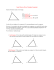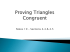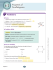# G.C.2 Wkst 5

## Transcription

G.C.2 Wkst 5
```G.C.2 STUDENT NOTES WS #5
1
Chord and Central Angle Properties
Theorem – In the same circle or in congruent circles,
if two chords are congruent then their minor arcs are
also congruent.
B
If….
C
AB = CD
AB ≅ CD
A
Converse of the Theorem – In the same circle or in
congruent circles, if two minor arcs are congruent,
then the chords are congruent.
B
If…..
C
Then….
E
D
AB ≅ CD
AB ≅ CD
A
Then….
E
D
mAB = mCD
Proof of Theorem
mAB = mCD
AB = CD
AB ≅ CD
Proof of the Converse of the Theorem
Given: AB ≅ CD
B
B
Given: AB ≅ CD
C
C
A
A
EA ≅ EB ≅ EC ≅ ED because
form two congruent ∆’s with the
given by SSS. Thus the central
angles ∠AEB ≅ ∠DEC
establishing that the arcs that
they subtend are also congruent,
E
D
EA ≅ EB ≅ EC ≅ ED because
∠AEB ≅ ∠DEC because they
are central angles on the
congruent arcs. This creates
two ∆’s that are congruent by
SAS. The congruent ∆’s
establish that AB ≅ CD .
θ
E
θ
D
AB ≅ CD .
EXAMPLES
Find m CF = _____
Find x = ______
Find m CE = _______
F
x
C
x
E
8.5 cm
108°
A
C
A
x
8.5 cm
A
10 cm
E
D
106°
x = 360 - 106 – 106 – 33 = 115°
F
2 cm
D
Equal arcs = Equal chords x = 10 cm
x = 360 - 108 = 252/3 = 84°
Theorem – If a diameter of a circle is perpendicular to
a chord, then the diameter bisects the chord and its
arc.
Converse of the Theorem – If a chord is a
perpendicular bisector of another chord, then the
first chord is a diameter.
E
E
If diameter BE is ⊥ to a chord,
If BE is the perpendicular
then AB ≅ BC ( AB = BC )
bisector of AC , then BE is
a diameter.
D
And AF ≅ FC ( mAF = mFC )
A
2 cm
8.5 cm
33°
B
F
C
A
B
C
G.C.2 STUDENT NOTES WS #5
2
Proof of the Theorem
Proof of Converse of the Theorem
E
Given that If BE is a diameter that
is perpendicular to a chord.
DA ≅ DC because they are radii
D
and DB ≅ DB because they are
common. ∆ADB ≅ ∆CDB by HL.
C
B
F
A
The perpendicular bisector of AC must go
through the center because it represents all
points that are equidistant from A and C and the
center is by definition equidistant to all points on
the circle.
Thus making AB ≅ BC and central
congruent arcs.
EXAMPLES
Find x = _____
Find x = _____ (2 dec.)
Find x = ______
x
x
13 cm
A
A
4 cm
x
4 cm
10 cm
5 cm
3 cm
1 cm
52 + y2 = 132 y= 12 cm
d = 12 + 1 = 13 cm x = 6.5 cm
42 + 52 = x2 x ≈ 6.40 cm
Theorem – If two chords are equidistant from the
center, then they are congruent.
If GE ≅ GF and
A
Converse of the Theorem – In the same circle, or in
congruent circles, if two chords are congruent, then
they are equidistant from the center.
If AB ≅ CD
A
GE ⊥ AB and
E
E
G
B
F
D
C
GF ⊥ CD ,
then
AB ≅ CD
A
G
B
F
C
Then
G
B
F
D
GE ≅ GF
C
Proof of the Theorem
E
42 + 32 = x2 x ≈ 5 cm
D
The 4 ≅ radii and the given
help us to establish that ∆AEG
≅ ∆BEG ≅ ∆CFG ≅ ∆DFG by HL.
The congruent ∆’s provide
congruent sides and so
AB ≅ CD (AB = CD).
Proof of the Converse of the Theorem
The 4 ≅ radii and the given
A
help us establish congruent
E
G
∆’s by SSS. The two congruent
isosceles ∆’s must have ≅
B
F
D
heights. Thus they are the
C
same distance, GE ≅ GF .
G.C.2 STUDENT NOTES WS #5
3
EXAMPLES
Find x = _______
Find x = _______ (E)
Find x = _______
6.5 cm
x
A
A
12 2
x
x
A
4 cm
90°
x = 2(6.5) = 13 cm
45 – 45 - 90 x = 4 2 cm
45 – 45 - 90 x = 12 cm
G.C.2 WORKSHEET #5
Name: ________________________________ Period ______
1. Determine the requested value.
a)
b) IJ = 10 cm, HA = 11 cm
I
c)
1
d)
78°
55°
x
H
220°
25°
J
x
140°
9 cm
91°
10 cm
x
60°
B
27°
11 cm
12 cm
A
70°
113°
K
x = ___________
e)
AK = ___________
f)
x = ___________
h)
x
x = ___________
i)
3.5 cm
88°
x
x
74°
x = ___________
x = ___________
2. Determine the length of radius AC .
a)
b)
x = ___________
x = ___________
c)
d)
AC = __________ (2 dec.)
AC = __________ (E)
c)
d)
C
A
5 cm
24 cm
AC = __________
B
AC = __________ (2 dec.)
3. Determine the requested value.
a)
b)
20 cm
5 cm
x = ___________
x = ___________
x = ___________ (E)
x
x = ___________ (E)
G.C.2 WORKSHEET #5
2
4. Determine the requested value. Some of these questions are quite… “SPECIAL”.
a)
b)
c)
d)
x = ______________ (E)
x = ______________ (E)
x = ______________ (E)
x = ______________ (E)
e)
f)
g)
h)
x = ______________
x = ______________
B
C
A
AB = ______________ (E)
x
x = ______________
5. A student questions the teacher…. “It makes sense that if you have congruent
chords you would have congruent arcs but can you prove it?” Help the teacher by
proving this to be true.
D
C
E
B
A
6. Why would the perpendicular bisector of a chord have to be a diameter?
7. An ancient plate from the Mayan time period was drop at a museum. The
curator wanted to put it back together but needed to find the center of the
place to reference the restoration. If the largest piece looked like this… how
could they find the center of the plate?
(o
uo^",
6.C.2 WORKSHEET #5
I
I
Y
period
1. Determine the requested value.
b) lJ = 10 cm, HA = 11cm
a)
-0
x=
lLc^^
x=
e)
x=
h)
Yv
x=
1c^
i)
q,v
r
bL-O
*4
x= I cu
x=
2. Determine the tength of radius AC
a)
x=
.
b)
A
4'
AC=
L,s?+
13 c't"
AC=
(2
dec.)
AC =
3
,to
cm
f=*-
(2 dec.)
AC=
(E)
3. Determine the requested value.
*= 2(
(A
x=
x= 66
crx
(E)
(E)
G.C.2 WORKSHEET #5
2
4. Determine the requested value. Some of these questions are quite... ,SPEC|AL".
x=
sG cA (E)
f)
e)
AB
(E)
x=
x=
11"^
q^
x=
(E)
h)
8)
= [email protected] Crr (E) x=
x=
(E)
lL cw
x=
5. A student questions the teacher....
"!t makes sense that if you have congruent
chords you would have congruent arcs but can you prove it?,, Help the teacher by
proving this to be true.
Dvo.,:
in
red:i ,
; ,6
, eu
,*
A Ctp U A ffg, €s)
/- Cev E LD7A P e-ca^s*- AZ.A
,/
v*Y gou"\te''\
6s,'n1v
"-artjLn-s -'z ,*ln:*
5. why would the perpendicular bisector of a chord have to be a diameter?
7. An ancient plate from the Mayan time period was drop at a museum. The
curator wanted to put it back together but needed to find the center of the
place to reference the restoration. lf the largest piece looked like this... how
could they find the center of the plate?
&YL),
!.)^
(
(
```

### 12-2 Practice Chords and Arcs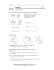### Geometry Secondary Education MAFS.912.G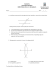### HW 1 - Solutions to selected exercises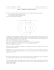### C Geometry Name: 1.4 Worksheet – Constructing Parallel and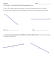### Geometry 4.7 worksheet Name Period _____ 1. Sketch the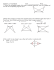### Geometry Practice Quiz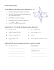### Root Position 3-Note Chords www.JasonWerkema.com (for 6-string Bass)### Some Ways to Prove Triangles Congruent